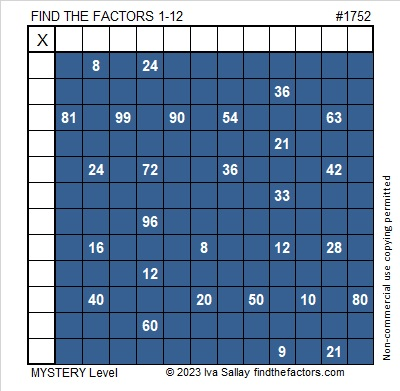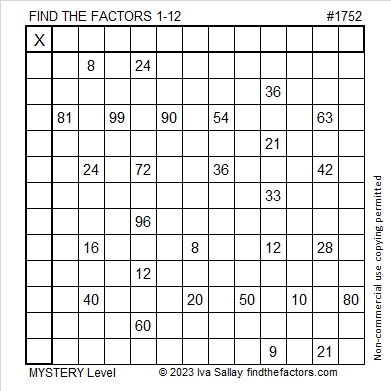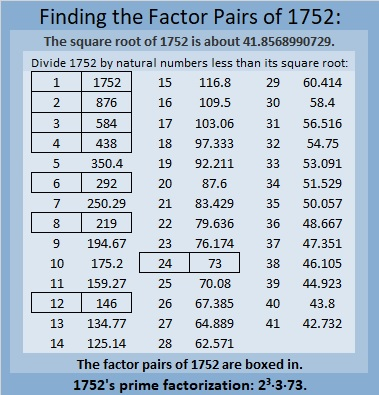# 1752 So Many Snowflakes

Contents

### Today’s Puzzle:

A winter storm hit much of the United States today including the drought-stricken area where I live. We are so grateful for the snow, but also grateful we could stay home all day. Driving in this much snow is not much fun.

This snowflake mystery-level puzzle might have a couple of tricky clues, but I’m confident that you’ll still find it easier than clearing a couple of feet of snow off a driveway! Just write the numbers 1 to 12 in both the first column and the top row so that those numbers and the given clues make a multiplication table.Here’s the same puzzle without any added color:### Factors of 1752:

• 1752 is a composite number.
• Prime factorization: 1752 = 2 × 2 × 2 × 3 × 73, which can be written 1752 = 2³ × 3 × 73.
• 1752 has at least one exponent greater than 1 in its prime factorization so √1752 can be simplified. Taking the factor pair from the factor pair table below with the largest square number factor, we get √1752 = (√4)(√438) = 2√438.
• The exponents in the prime factorization are 3,1 and 1. Adding one to each exponent and multiplying we get (3 + 1)(1 + 1) (1 + 1) = 4 × 2 × 2 = 16. Therefore 1752 has exactly 16 factors.
• The factors of 1752 are outlined with their factor pair partners in the graphic below.### More About the Number 1752:

1752 is the hypotenuse of a Pythagorean triple:
1152 1320 1752, which is 24 times (48-55-73).

1752 is the difference of two squares in FOUR different ways:
439² – 437² = 1752,
221² – 217² = 1752,
149² -143² = 1752, and
79² – 67² = 1752.

1752 is a palindrome in some other bases:
It’s A4A in base13 because 10(13²) + 4(13) + 10(1) = 1752,
it’s 4G4 in base19 because 4(19²) + 16(19) + 4(1) = 1752, and
it’s 2K2 in base 25 because 2(25²) + 20(25) + 2(1) = 1752.

This site uses Akismet to reduce spam. Learn how your comment data is processed.